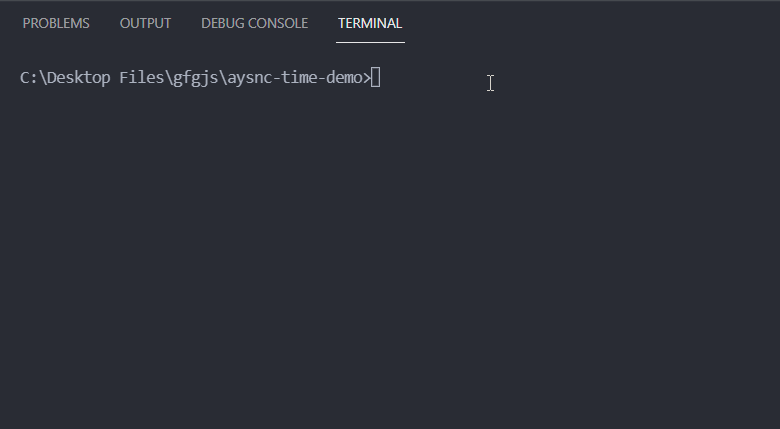Open in App
Not now

# Measure the duration of async operations in Node.js

• Last Updated : 22 Oct, 2021

Asynchronous operation in Node.js is a non-blocking operation which means if we perform an asynchronous operation at a certain point in code then the code after that is executed and does not wait for this asynchronous operation to complete.

If we want to calculate the duration of an asynchronous operation then we can do it easily inside of our async function using the syntax mentioned below.

Syntax:

```const calcTime = async () => {
const start = Date.now();
await someAsyncOperation();
const end = Date.now()
const duration = end - start;
}```

Project Setup:

Step 1: Install Node.js if you haven’t already.

Step 2: Create a folder for your project and cd (change directory) into it. Create a new file named app.js inside that folder.

Project Structure: After following the steps your project structure will look like the following.In the code example mentioned below, we have simulated an asynchronous operation using setTimeout() method. We perform the addition operation inside an async function that returns a promise. This promise is resolved with the result of addition after 2 seconds. Finally, we calculate and display the time taken to perform the addition operation inside our IIFE (Immediately Invoked Function Expression).

## app.js

 `const asyncAdd = async (a, b) => {``  ``return` `new` `Promise((resolve, reject) => {``    ``setTimeout(() => {``      ``resolve(a + b);``    ``}, 2000);``  ``});``};`` ` `(async() => {``  ``const startTime = Date.now();``  ``const res = await asyncAdd(5, 2);``  ``const endTime = Date.now();`` ` `  ``const timeTaken = endTime - startTime;`` ` `  ``console.log(`Result of addition = \${res}`);``  ``console.log(`Time taken to perform addition =``          ``\${timeTaken} milliseconds`);``})();`

Step to run the application: We can execute our app.js file using the following command on the command line.

`node app.js`

Output:My Personal Notes arrow_drop_up